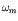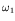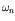Computational & Technology Resources an online resource for computational,engineering & technology publications not logged in - login Civil-Comp ProceedingsISSN 1759-3433 CCP: 83PROCEEDINGS OF THE EIGHTH INTERNATIONAL CONFERENCE ON COMPUTATIONAL STRUCTURES TECHNOLOGY Edited by: B.H.V. Topping, G. Montero and R. Montenegro Paper 143Evaluation of the Damping Matrix and Its Effect on the Dynamic Response of Suspension Bridges S. Esteki1 and S. Pourzeynali21Department of Civil Engineering, Faculty of Engineering, The Islamic Azad University of Shahr-e-Kord, I.R. Iran 2Department of Civil Engineering, Faculty of Engineering, The University of Guilan, Rasht, I.R. Iran doi:10.4203/ccp.83.143 Full Bibliographic Reference for this paper S. Esteki, S. Pourzeynali, "Evaluation of the Damping Matrix and Its Effect on the Dynamic Response of Suspension Bridges", in B.H.V. Topping, G. Montero, R. Montenegro, (Editors), "Proceedings of the Eighth International Conference on Computational Structures Technology", Civil-Comp Press, Stirlingshire, UK, Paper 143, 2006. doi:10.4203/ccp.83.143 Keywords: damping matrix, Rayleigh method, TMD system, suspension bridges. Summary In structural dynamics different formulations are suggested for construction of viscous damping matrices among which the Rayleigh method can be mentioned. In most structures, distribution of the damping mechanism throughout the structure is uniform, hence one can assume that the damping matrix is proportional to either mass or stiffness matrix of the structure. A widely used solution for this problem was suggested by Lord Rayleigh, in which the damping matrix is assumed to be proportional to both mass and stiffness matrices. No specific combination of mass and stiffness matrices is recommended for the construction of the damping matrix. Since, accuracy of the results might be affected by such assumption, therefore, accurate evaluation of the damping matrix [C] is essential. Calculation of [C] is not possible using geometric and mechanical properties of the structure. Therefore, it becomes the most popular strategy among the structural engineers to assume the values of modal damping ratios for some specific modes and then calculate the [C] matrix using the Rayleigh method. Evidently, the response of the structures directly depends on the assumed values of the modal damping ratios. Suspension bridges due to their long spans could be very susceptible to vertical vibrations caused by earthquake vertical acceleration components. Therefore, evaluation of the accurate response of these bridges depends on the damping ratios assumed in the analyses. As well, the approximated damping ratios could affect the performance of the tuned mass damper (TMD) control system used in this study to suppress the vertical response of the bridge. In this paper, for calculating the damping matrix from Rayleigh method, different combinations of mass and stiffness matrices are used in the dynamic finite element analysis of suspension bridges strengthened by TMD systems. The effects of the damping matrices obtained on the dynamic response of the suspension bridges are examined and the accuracy of the results compared. As well, the effect of the calculated damping matrix on uncontrolled and controlled (by TMD) responses of the suspension bridges is investigated. The Thomas Suspension Bridge, located in Los Angeles, California, USA, is chosen as the numerical example. The equation of motion of the system is written using the finite element method and energy principles. The system is subjected to earthquake vertical acceleration. The bridge deck is modelled as a simply supported beam connected by several hangers to the main cables. In the finite element model of the bridge, two node beam elements with two degrees of freedom at each node, vertical displacement and bending rotation, are considered. The bridge element consists of the bridge girder, at least two vertical hangers, and the main cables. The stiffness matrix of each element can be derived through calculation of the potential energy of the element, using the Hamilton's principle. The total stiffness matrix of the bridge, then, can be obtained by assembling the elemental stiffness matrices. The central goal in this paper is to find the best combination of the bridge vibrational modes to obtain the reasonable values of the modal damping ratios for all other modes of the bridge The following results are obtained from the numerical analyses: (a) in the Rayleigh method, in which two vibrational modes are selected to evaluate the proportionality coefficients, if the first mode () is set to the fundamental mode frequency (i.e., to) and the second mode () is set to the median (50 percentage) mode frequency, then it provides reasonable modal damping ratios for all modes; and (b) in the Rayleigh method, ifandboth are set to the lower mode frequencies, then the TMD control system does not have any effectiveness on the suppression of the suspension bridge maximum displacement, while whenis set to the, andis set to the higher mode frequency, then effectiveness of the TMD control system, from a reasonable prediction of the bridge modal damping ratios, is much better than the first case. purchase the full-text of this paper (price £20) Back to top ©Civil-Comp Limited 2023 - terms & conditions